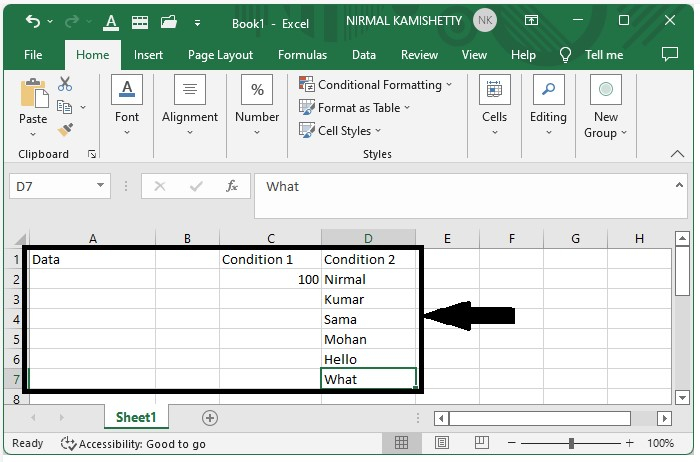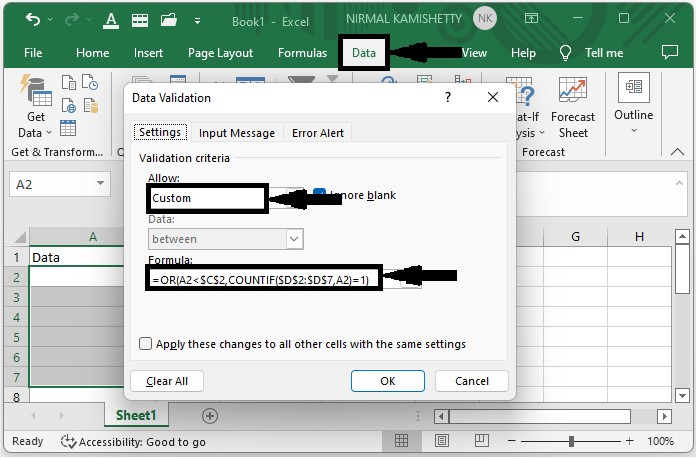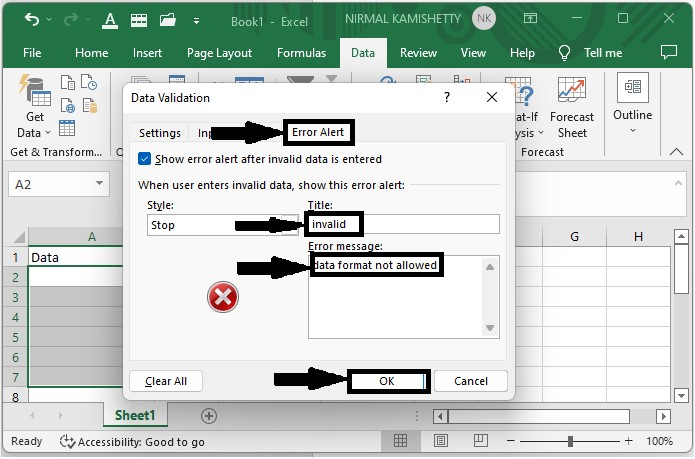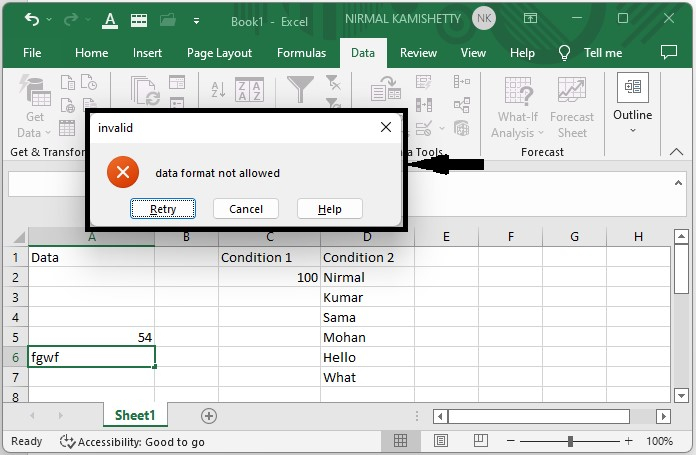# How to Apply Multiple Data Validation in One Cell in an Excel Worksheet?

Applying a single data validation to the cells in Excel is a very simple and frequently used process, but have you ever tried applying multiple data validations to a single cell at the same time? Read this tutorial to learn a simple way to apply multiple data validation rules in one cell in an Excel worksheet.

## Applying Multiple Data Validation in One Cell

We will use a single formula to perform multiple data validations. Let's look at a quick way to apply multiple data validations in one cell in Excel. The problem we solve in this article is that we only accept a number less than 500 or a text if it is present in the list provided in the Excel file. Our formula will contain both conditions.

Step 1

Assume we have an Excel sheet with data that looks like the image below.Step 2

Now to apply the data validation, select the range of cells where you want to apply the data validation, then click on data and click on data validation, and a new pop-up window will be opened as shown in the below image.Now in the new pop-up, select Allow as Custom and enter the formula =OR(A2<$C$2,COUNTIF($D$2:$D$7,A2)=1) in the formula box.

Step 3

Now to customise the error message, click on "Error alert," then enter Title as "Invalid" and Message as "data format not allowed" and click "OK" as shown in the below screenshot.Step 4

When we try to enter any other data, an error message will be displayed, as shown in the below image.## Conclusion

In this tutorial, we used a simple example to demonstrate how we can apply multiple data validations in one cell in Excel to highlight a particular set of data.